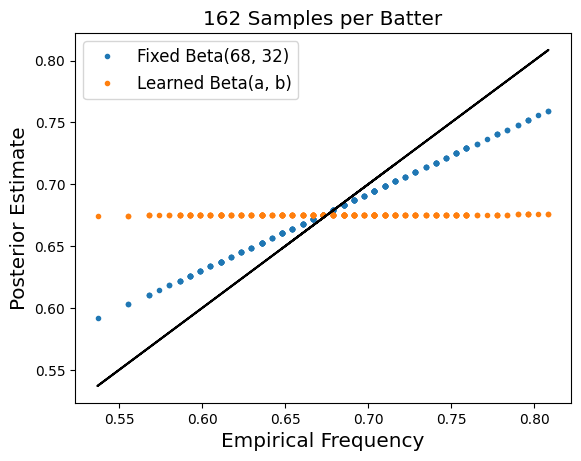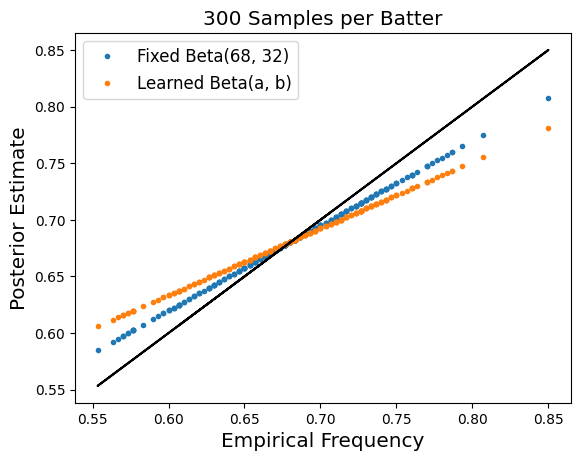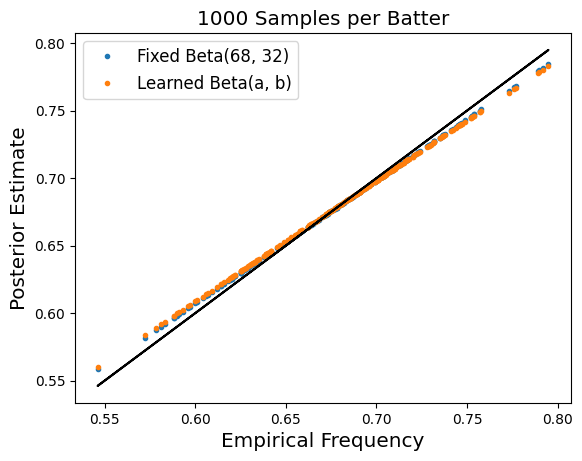### Beat the Streak: Day 11

In a previous blog post, I showed that simply looking at empirical frequencies to estimate hit probabilities can be misleading, as there is a positive bias that is introduced when we take the maximum over a bunch of empirical frequencies.  This bias will incorrectly lead us to believe that the probability of a hit for the best batter is higher than it truly is, which is clearly a problem from the perspective of beat the streak.

It is fairly straightforward to correct for the bias.  In this blog post, I will explain how, and discuss the implications of the bias-corrected hit probabilities.  Recall from the previous blog post, that our setup is as follows:

Suppose we have a collection of batters $i=1, \dots, 250$, and each batter has a certain (unknown) probability of getting a hit in a given game $p_i$.  Moreover, assume each batter plays in $162$ games, and that the outcomes for each player across games is i.i.d.  For the purposes of this problem abstraction, let's assume $p_i \sim Beta(68, 32)$.  Our observation is $n_i \sim Binom(162, p_i)$, and we'd like to estimate $p_i$ for each batter $i$.

In the absence of prior information  information $n_i / 162$ is the maximum likelihood estimator for $p_i$.  However, if we incorporate our prior information that $p_i \sim Beta(68, 32)$ it turns out that the maximum likelihood estimator is $p_i = \frac{68 + n_i}{162 + 68 + 32}$.  This new "posterior estimate" for $p_i$ regresses the estimate towards the mean value of $0.68$.  The plot below shows how the posterior estimate relates to the empirical frequency.  In particular, while the empirical frequency is as large as 0.833, the corresponding posterior estimate is only 0.777.  While this posterior estimate is lower, it more accurately reflects the true underlying probability of a hit.

There are two ways in which I would like to generalize the analysis above to better understand this problem.  (1) We can treat treat the parameters of the beta distribution (68 and 32 above) as learnable parameters rather than known quantities.  (2) we will look at how this trend changes as a function of the number of data points that we sample for each batter.  The three plots below demonstrate what happens when we make these two modifications to our setup:From the above plots, we make the following observations:

1. When we treat the Beta parameters as learnable, the resulting posterior estimates end up being more strongly regularized.  When there are only 162 samples per batter, the regularization strength is so strong that the estimated probabilities for each batter is very close to the mean of $0.68$.  Note that this is the maximum likelihood estimator given the observed data.

2. As we increase the number of samples per batter, the posterior estimates tend towards the empirical frequencies.  There is still a significant gap even with 300 samples per batter, but the gap is significantly closed at 1000 samples per batter.

The observations we made above are worthy of further discussion.  The left-most plot corresponds to the setting where we observed 1 years worth of data.  As we can see from the orange line, this is not really enough data by itself to make conclusive statements about players hit probabilities with high confidence.  If we know the Beta parameters in advance, we can do better, however, and we generally should be able to get those estimates dialed in pretty nicely using multiple years worth of data.

These plots suggest to me that it is not sufficient to look at game-level granularity data, since $162$ data points is simply not enough.  Working directly in terms of atbat-level (or even pitch-level) data, where we have access to roughly 4X samples per batter should be able to provide a much stronger signal for us.

## Analyzing Real Data

Thus far, we have studied this idea in theory on synthetically constructed data.  What if instead we feed in real data to estimate both the optimal beta parameters, as well as the maximum a-posteriori hit probabilities for each player?  We organize the data so that $n_i$ corresponds to the number of hits for a given i = (player, year), and $N_i$ denotes the number of games started for that (player, year) combo.  We filter out data points where $N_i < 100$.  Doing this,we find that the optimal beta parameters are $1900$ and $964$ respectively, which gives the following prior distribution over hit probabilities:

This is a much more concentrated distribution than we assumed in our idealized setting, and it suggests that the hit probability is rarely above 70%.  This is more or less in line with our experiment earlier in the blog post, that found when we learn the Beta(a, b) parameters for $N_i = 162$ the resulting hit probability estimates are nearly constant.  Here, they are not quite constant, but are still highly concentrated to the range 65%-68%.  Now we instead organize the data so that $N_i$ and $n_i$ correspond to the number of games played and games with hit respectively for a player $i$, aggregating across all years.  For the subset of players we consider, the average value of $N_i$ is $477$, much larger than in the single season setting.  Repeating the same experiment from earlier finds the optimal Beta parameters are $80$ and $47$ respectively, which gives us the following prior distribution over hit probabilities.

Moreover, the posterior estimate of the hit probability for the top $5$ batters according to this model are shown below.  Comparing to the empirical hit frequency, it is slightly regularized (more so for the batters with fewer seasons worth of data, like Trea Turner).  The estimated hit probabilities are higher than they were when we only looked at a single season's worth of player data at a time, which is encouraging.  However, the magnitude of both the empirical hit frequencies and posterior estimated probabilities is not really large enough for any of these batters to beat the streak.

batter Posterior Estimated Hit Probability
Charlie Blackmon 0.733585
Michael Brantley 0.734289
Daniel Murphy 0.736962
Jose Altuve 0.740695
Trea Turner 0.754412

batter Hit Frequency
Daniel Murphy 0.749113
Jose Altuve 0.749839
Billy Burns 0.752577
Scott Podsednik 0.758242
Trea Turner 0.774074